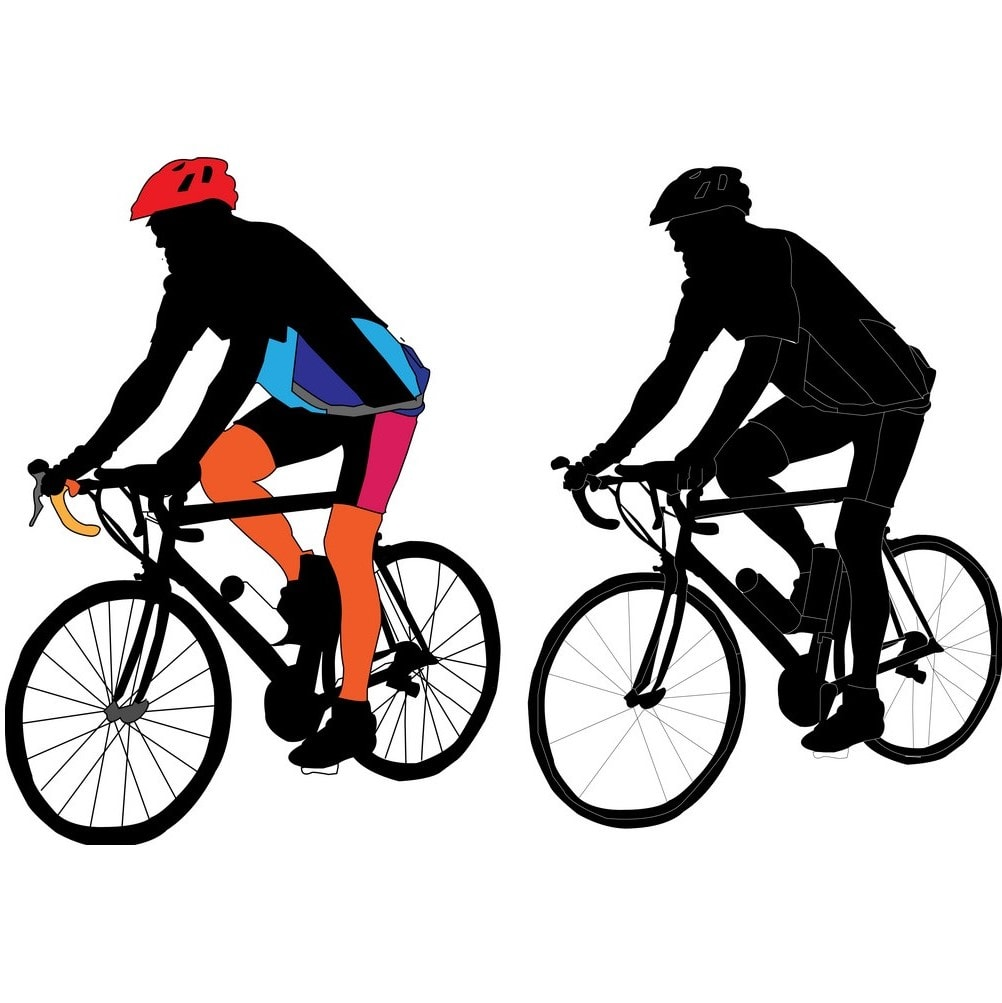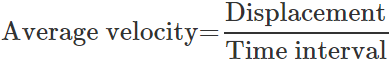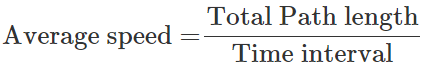Courses

# Short & Long Answer Question - Motion in a Straight Line Class 11 Notes | EduRev

## NEET : Short & Long Answer Question - Motion in a Straight Line Class 11 Notes | EduRev

The document Short & Long Answer Question - Motion in a Straight Line Class 11 Notes | EduRev is a part of the NEET Course Physics For JEE.
All you need of NEET at this link: NEET

Q.1 When does a cyclist appear to be stationary with respect to another moving cyclist?

Answer: When both the cyclists are moving in the same direction with the same velocity parallel to each other.Two Cyclist with same SpeedQ.2 Can the earth be regarded as point object when it is describing its yearly journey around the sun?

Answer: Yes, because size of the earth is much smaller than the distance from the sun.
Q.3 Can the displacement be greater than the distance travelled by an object? Give reason.

Answer: No, the displacement of an object can be either equal to or less than the distance travelled by the object. This is because displacement is shortest distance between the initial and final positions of the object while distance travelled is the length of the actual path traversed by the object.
Q.4 Can the speed of a body be negative?

Answer: No, because the speed of an object is the distance travelled per unit time and distance travelled is never negative.
Q.5 Can a body have a constant speed and still have a varying velocity?

Answer: Yes, a particle in uniform circular motion has a constant speed but varying velocity because of the change in its direction of motion at every point.
Q.6 Can a body have a constant velocity and still have a varying speed?

Answer: No, a body cannot have a constant velocity while having a varying speed. Whenever the speed changes, velocity also changes.
Q.7 Can a particle in one-dimensional motion have zero speed and a non-zero velocity?

Answer: No, if the speed is zero, the velocity will be necessarily zero.
Q.8 Can a body have zero velocity and still be accelerating?

Answer: Yes, a body thrown vertically upwards has zero velocity at its highest point but has acceleration equal to the acceleration due to gravity.
Q.9 Can an object have an eastward velocity while experiencing a westward acceleration?

Answer: Yes, a pendulum oscillating in east-west direction will have eastward velocity and westward acceleration in half cycle of its oscillation.

Q.10 Can the direction of velocity of an object change, when acceleration is constant?

Answer: Yes, for an object thrown vertically upwards, the direction of velocity changes during its rise and fall. But acceleration acts always downwards and remains constant.
Q.11 Is it possible for a body to be accelerated without speeding up or slowing down? If so, give an example.

Answer: Yes, an object in uniform circular motion is accelerating but its speed neither decreases nor increases.
Q.12 Under what condition is the average velocity equal to the instantaneous velocity?

Answer: When a body moves with a constant velocity, its average velocity over any time interval is same as is instantaneous velocity.
Q.13 Why is the speed, in general, greater than the magnitude of the velocity?

Answer: Because of the change in the direction of motion, the length of the path traversed by a body is generally greater than the magnitude of its displacement. So speed is generally greater than the magnitude of velocity.
Q.14 Is the direction of acceleration same as the direction of velocity?

Answer: Not necessarily. If velocity increases, acceleration acts in the direction of velocity and if velocity decreases, then acceleration acts in the opposite direction of velocity.
Q.15 Can we use the equations of kinematics to find the height attained by a body projected upwards; with any velocity?

Answer: No, the equations of kinematics are  applicable only so long as the acceleration is uniform. The acceleration due to gravity is uniform only near the surface of the earth.
Q.16 Two balls of different masses (one lighter and other heavier) are thrown vertically upward with same initial speed. Which one will rise to the greater height?

Answer: Both the balls will rise to the same heights. It is because, for a body moving with given initial velocity and acceleration, the distance covered by the body does not depend on the mass of the body.
Q.17 Two balls of different masses (one lighter and other heavier) are thrown vertically upwards; with the same speed. Which one will pass through the point of projection in their downward direction with the greater speed?

Answer: In case of motion under gravity, the speed with which a body returns back is always equal to the speed with which it is thrown up. Since expression for final speed does not involve mass, both the balls will acquire the same speed.

Q.18 Can the relative velocity of two bodies be greater than the absolute velocity of either body?

Answer: Yes, when two bodies move in opposite directions, the relative velocity of each is greater than the individual velocity of either body.
Q.19 A car travelling with a velocity of 50 kmh-1  on a straight road is ahead of a motor-cycle travelling with a speed of 75 kmh-1 . How would the relative velocity be altered if motor cycle is ahead of car?

Answer: The relative velocity will remain same as it does not depend on the position of the two bodies.
Q.20 Even when rain is falling vertically downwards, the front screen of a moving car gets wet while the back screen remains dry. Why?

Answer: This is because the rain strikes the car in the direction of relative velocity of rain with respect to car.
Q.21 Is it possible that the brakes of a car are so perfect that the car stops instantaneously. If not, why?

Answer: No, it is not possible. In order to make velocity zero in an infinitesimally small interval of time, the car needs an infinite declaration which is not possible.
Q.22 When an observer is standing on earth, the trees and houses appear stationary to him. However, when he is sitting in a moving train, all these objects appear to move in backward direction. Why?

Answer: For the stationary observer, the relative velocity of trees and houses is zero. For the observer sitting in the moving train, the relative velocity of houses and trees is negative. So these objects appear to move in backward direction.
Q.23 The average velocity of a particle is equal to its instantaneous velocity. What is the nature of its displacement-time graph?

Answer: When the average velocity is equal to the instantaneous velocity, the particle has a uniform motion. So its displacement-time graph is a straight line inclined to the time-axis.
Q.24 Can the position-time graph have a negative slope?

Answer: Yes, when the velocity of the object is negative.
Q.25 What is the nature of the displacement- time curve of a body moving with constant velocity?

Answer: Parabola.
Q.26 Can the direction of motion of a body change if its velocity is changing at uniform rate?

Answer: Yes. This can happen in a retarded motion. A body thrown up moves under constant retardation and from the highest point it begins to fall downwards.
Q.27 Is it possible to have a constant rate of change of velocity when velocity changes both in magnitude and direction? If yes, give one example.

Answer: Yes, in projectile motion, a body has uniform acceleration in the downward direction while its velocity changes both in magnitude and direction at every point of its trajectory.
Q.28 Can a body be at rest as well as in motion at the same time? Explain. Or Rest and motion are relative terms. Explain.

Answer: Yes, object may be at rest relative to one object and at the same time it may be in motion relative to another object. For example, a passenger sitting in a moving train is at rest with respect to his fellow passengers but he is in motion with respect to the objects outside the train. Rest and motion are relative terms.
Q.29 If the displacement of a body is zero, is the distance covered by it necessarily zero? Comment with suitable illustration.

Answer: No, it is not necessary that the distance covered by a body is zero when its displacement is zero. Consider a particle moving along a circular track of radius r. After the particle completes one revolution, its displacement is zero while the distance covered is 2πr.
Q.30 State in the following cases, whether the motion is one, two or three dimensional: (i)a kite flying on a windy day, (ii) a speeding car on a long straight high way, (iii) a carrom coin rebounding from the side of the board, (iv) an insect crawling on a globe, and (v) a planet revolving around its star ?

Answer: (i) three-dimensional,    (ii) one-dimensional, (iii) two-dimensional, (iv) two-dimensional, (v) two-dimensional.
Q.31 Which of the two-velocity or acceleration, gives the direction of motion of the body. Justify your answer by an example.

Answer: It is the velocity and not the acceleration, which gives the direction of motion of a body. When a body is projected upward, both its direction of motion and velocity are in upward direction but the acceleration is in the downward direction.
Q.32 Show that the average velocity of an object over an interval of time is either smaller than or equal to the average speed of the object over the same interval.
Answer:
We know thatAs the magnitude of the displacement of an object can be equal to or less than the total length of the path traversed by the body in given time, so average velocity of an object over an interval of time is either equal to or smaller than the average speed of the object over the same interval.

Q.33 Distinguish between speed and velocity.
Answer:
Speed Velocity

1. It is the distance travelled by a body per unit time in any direction. It is the distance travelled by a body per unit time in a fixed direction.

2. It is a scalar quantity. It is a vector quantity.

3. Speed may be positive or zero but never negative. Velocity may be positive, negative or zero.

Offer running on EduRev: Apply code STAYHOME200 to get INR 200 off on our premium plan EduRev Infinity!

## Physics For JEE

188 videos|346 docs|307 tests

,

,

,

,

,

,

,

,

,

,

,

,

,

,

,

,

,

,

,

,

,

;